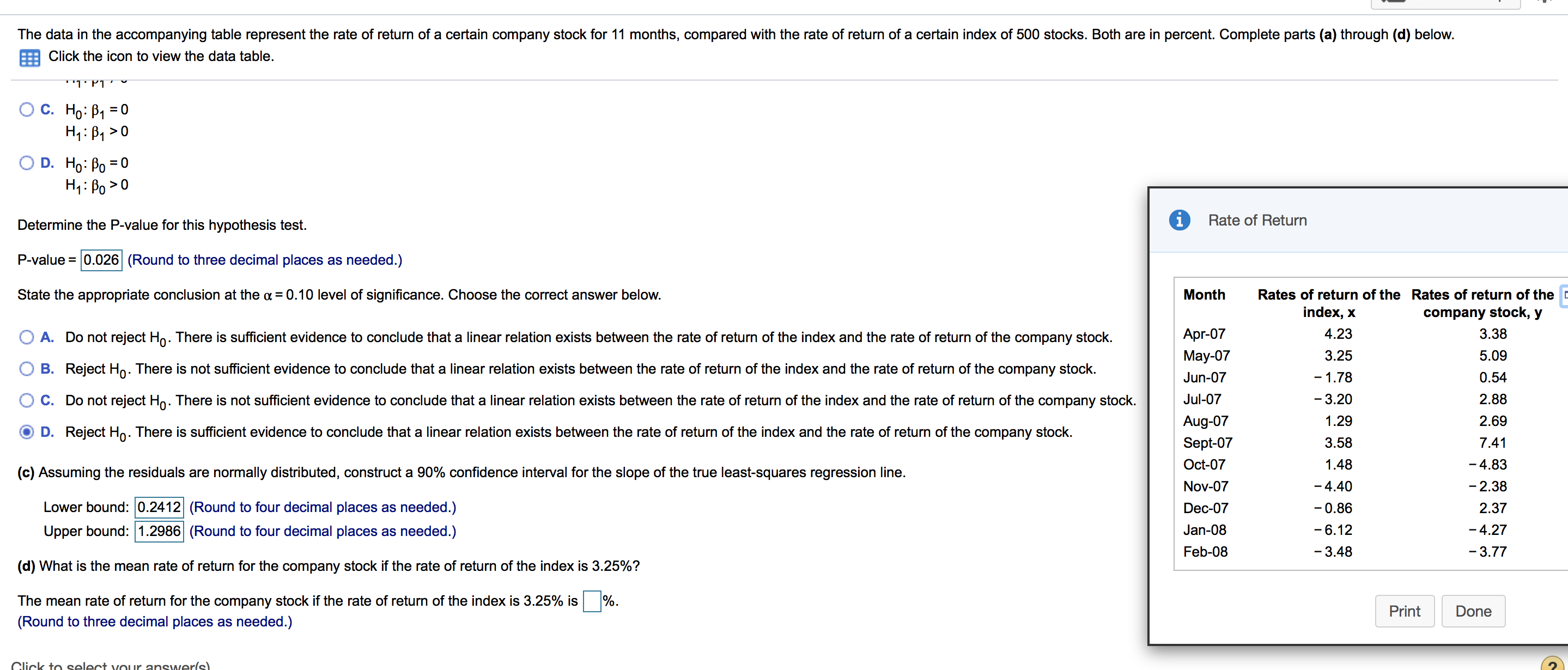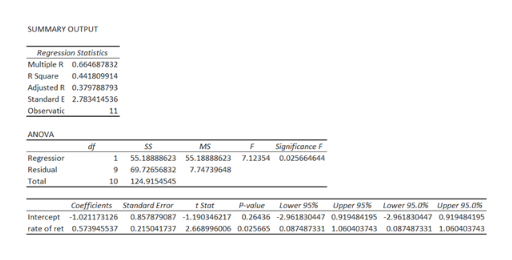# The data in the accompanying table represent the rate of return of a certain company stock for 11 months, compared with the rate of return of a certain index of 500 stocks. Both are in percent. Complete parts (a) through (d) below.Click the icon to view the data table''l' P1.ΤΡΤС. Но: В1 %3D0O D. Ho: Po 0Rate of ReturnDetermine the P-value for this hypothesis test.P-value 0.026 (Round to three decimal places as needed.)State the appropriate conclusion at the a =0.10 level of significance. Choose the correct answer below.MonthRates of return of the Rates of return of theindex, xcompany stock, yApr-074.233.38return of the company stock.A. Do not reject Ho. There is sufficient evidence to conclude that a linear relation exists between the rate of return of the index and the rate3.25May-075.09B. Reject H. There is not sufficient evidence to conclude that a linear relation exists between the rate of return of the index and the rate of return of the company stock.-1.780.54Jun-072.88C. Do not reject Ho. There is not sufficient evidence to conclude that a linear relation exists between the rate of return of the index and the rate of return of the company stockJul-07-3.20Aug-071.292.69D. Reject Ho. There is sufficient evidence to conclude that a linear relation exists between the rate of return of the index and the rate of return of the company stock.Sept-073.587.41Oct-071.48-4.83(c) Assuming the residuals are normally distributed, construct a 90% confidence interval for the slope of the true least-squares regression line- 4.40- 2.38Nov-07Lower bound: 0.2412 (Round to four decimal places as needed.)-0.86Dec-072.37Upper bound: 1.2986 (Round to four decimal places as needed.)Jan-08-6.12-4.27Feb-08-3.48-3.77(d) What is the mean rate of return for the company stock if the rate of return of the index is 3.25%?The mean rate of return for the company stock if the rate of return of the index is 3.25% isPrintDone(Round to three decimal places as needed.)Click to select vour answerls)

Question
48 views

I need help with the last question only. (d). thankshelp_outlineImage TranscriptioncloseThe data in the accompanying table represent the rate of return of a certain company stock for 11 months, compared with the rate of return of a certain index of 500 stocks. Both are in percent. Complete parts (a) through (d) below. Click the icon to view the data table ''l' P1. ΤΡΤ С. Но: В1 %3D0 O D. Ho: Po 0 Rate of Return Determine the P-value for this hypothesis test. P-value 0.026 (Round to three decimal places as needed.) State the appropriate conclusion at the a =0.10 level of significance. Choose the correct answer below. Month Rates of return of the Rates of return of the index, x company stock, y Apr-07 4.23 3.38 return of the company stock. A. Do not reject Ho. There is sufficient evidence to conclude that a linear relation exists between the rate of return of the index and the rate 3.25 May-07 5.09 B. Reject H. There is not sufficient evidence to conclude that a linear relation exists between the rate of return of the index and the rate of return of the company stock. -1.78 0.54 Jun-07 2.88 C. Do not reject Ho. There is not sufficient evidence to conclude that a linear relation exists between the rate of return of the index and the rate of return of the company stock Jul-07 -3.20 Aug-07 1.29 2.69 D. Reject Ho. There is sufficient evidence to conclude that a linear relation exists between the rate of return of the index and the rate of return of the company stock. Sept-07 3.58 7.41 Oct-07 1.48 -4.83 (c) Assuming the residuals are normally distributed, construct a 90% confidence interval for the slope of the true least-squares regression line - 4.40 - 2.38 Nov-07 Lower bound: 0.2412 (Round to four decimal places as needed.) -0.86 Dec-07 2.37 Upper bound: 1.2986 (Round to four decimal places as needed.) Jan-08 -6.12 -4.27 Feb-08 -3.48 -3.77 (d) What is the mean rate of return for the company stock if the rate of return of the index is 3.25%? The mean rate of return for the company stock if the rate of return of the index is 3.25% is Print Done (Round to three decimal places as needed.) Click to select vour answerls) fullscreen
check_circle

Step 1

Step-by-step procedure to obtain the regression equation using Excel:

• In Excel sheet, enter Rate of return of the index in one column and enter rate of return of the company stock in one column.
• In Data, select Data Analysis and choose Regression.
• In Input Variable X Range, select Rate of return of the index.
• In Input Variable Y Range, select rate of return of the company stock.
• Click Ok.

Output obtained using Excel is given below:help_outlineImage TranscriptioncloseSUMMARY OUTPUT Regression Stotistics Multiple R 0.664687832 R Square 0.441809914 Adjusted R 0.379788793 Standard E 2.783414536 Observatic 11 ANOVA Significance F df SS MS Regressior Residual 1 55.18888623 55.18888623 7.12354 0.025664644 7.74739648 9 69.72656832 Total 10 124.9154545 Coefficients Standard Error Upper 95% p-yalue Lower 95% Lower 95.0% Upper 95.0% t Stot Intercept -1.021173126 0.857879087 -1.190346217 0.26436 -2.961830447 0.919484195 -2.961830447 0.919484195 rate of ret 0.573945537 0.215041737 2.668996006 0.025665 0.087487331 1.060403743 0.087487331 1.060403743 fullscreen
Step 2

From the output, the regression equation is y^= –1.021+0.573x.

The mean rate of return f...

### Want to see the full answer?

See Solution

#### Want to see this answer and more?

Solutions are written by subject experts who are available 24/7. Questions are typically answered within 1 hour.*

See Solution
*Response times may vary by subject and question.
Tagged in

### Probability